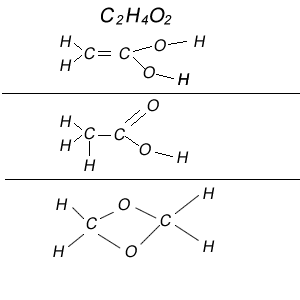Covalency and drawing molecules. The covalency of an atom is the number of electrons it shares with other atoms when forming chemical bonds. Usually, but not always, it is the number of covalent bonds it forms with other atoms.The periodic table always provides information about elements. Knowing the number of covalent bonds that each non-metal atom should form enables us to have a really good go at working out what a molecule should look like. 1) Let's start with our first example. Work out the molecular structure of the molecule with the formula C2H6 Step 1 Write down the covalency of each atom in the molecule, in other words the number of covalent bonds each atom can form. Step 2 Draw a line for each covalent bond Step 3 Draw the number of each atom present in the molecule. Step 4 Assemble the atoms so that each one has the right number of covalent bonds.2) Work out the molecular structure of the molecule with the formula C2H4 Step 1 Write down the covalency of each atom in the molecule, in other words the number of covalent bonds each atom can form. Step 2 Draw a line for each covalent bond Step 3 Draw the number of each atom present in the molecule. Step 4 Assemble the atoms so that each one has the right number of covalent bonds.2) Work out the molecular structure of the molecule with the formula C2H4O2 Step 1 Write down the covalency of each atom in the molecule, in other words the number of covalent bonds each atom can form. Step 2 Draw a line for each covalent bond Step 3 Draw the number of each atom present in the molecule. Step 4 Assemble the atoms so that each one has the right number of covalent bonds. Click to see a 3D representation of what the molecule might look like.Now there are many ways that a molecule with the formula C2H4O2 can have its atoms arranged and still have each atom with the right number of covalent bonds. Shown on the right are three different ways in which this could be achieved.1) Give a likely structure for each of the molecules with the following formulae. a) C2H6O b) N2O2H2 c) HNO d) CCl4 e) ClCOOH f) SH2 Solutions